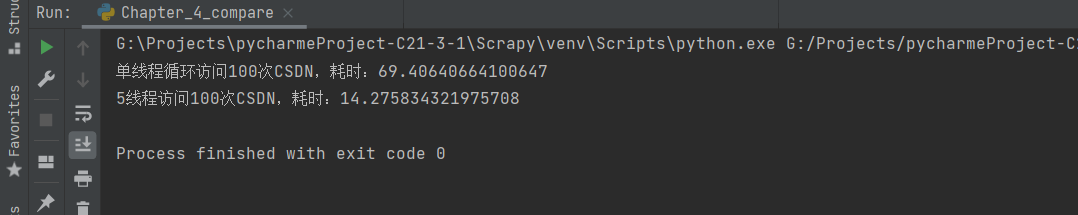## 前言

### 一、多进程库（multiprocessing）

`multiprocessing` 本身是`Python的多进程库`，用来处理与多进程相关的操作。但是由于进程与进程之间不能直接共享内存和堆栈资源，而且启动新的进程开销也比线程大得多，因此使用多线程来爬取比使用多进程有更多的优势。

multiprocessing下面有一个`dummy模块` ，它可以让Python的线程使用multiprocessing的各种方法

dummy下面有一个`Pool类` ，它用来实现线程池。这个线程池有一个`map()方法`，可以让线程池里面的所有线程都“同时”执行一个函数

计算0～9的每个数的平方

``````# 循环
for i in range(10):
print(i ** i)
``````

``````from multiprocessing.dummy import Pool

# 平方函数
def calc_power2(num):
return num * num

# 定义三个线程池
pool = Pool(3)
# 定义循环数
origin_num = [x for x in range(10)]
# 利用map让线程池中的所有线程‘同时’执行calc_power2函数
result = pool.map(calc_power2, origin_num)
print(f'计算1-10的平方分别为：{result}')
``````### 二、多线程爬虫

``````import time
import requests
from multiprocessing.dummy import Pool

# 自定义函数
def query(url):
requests.get(url)

start = time.time()
for i in range(100):
query('https://www.csdn.net/')
end = time.time()
print(f'单线程循环访问100次CSDN，耗时：{end - start}')

start = time.time()
url_list = []
for i in range(100):
url_list.append('https://www.csdn.net/')
pool = Pool(5)
pool.map(query, url_list)
end = time.time()
print(f'5线程访问100次CSDN，耗时：{end - start}')
``````### 三、案例实操

https://www.kanunu8.com/book2/11138/ 爬取`《北欧众神》`所有章节的网址，再通过一个多线程爬虫将每一章的内容爬取下来。在本地创建一个“北欧众神”文件夹，并将小说中的每一章分别保存到这个文件夹中，且每一章保存为一个文件。

``````import re
import os
import requests
from multiprocessing.dummy import Pool

# 爬取的主网站地址
start_url = 'https://www.kanunu8.com/book2/11138/'
"""

:param url: 网址
:return: 网页源代码
"""
def get_source(url):
html = requests.get(url)
return html.content.decode('gbk')  # 这个网页需要使用gbk方式解码才能让中文正常显示

"""

:param html: 目录页源代码
:return: 每章链接
"""
def get_article_url(html):
article_url_list = []
article_block = re.findall('正文(.*?)<div class="clear">', html, re.S)
article_url = re.findall('<a href="(\d*.html)">', article_block, re.S)
for url in article_url:
article_url_list.append(start_url + url)
return article_url_list

"""

:param html: 正文源代码
:return: 章节名，正文
"""
def get_article(html):
chapter_name = re.findall('<h1>(.*?)<br>', html, re.S)
text_block = re.search('<p>(.*?)</p>', html, re.S).group(1)
text_block = text_block.replace('&nbsp;', '')           # 替换 &nbsp; 网页空格符
text_block = text_block.replace('<p>', '')              # 替换 <p></p> 中的嵌入的 <p></p> 中的 <p>
return chapter_name, text_block

"""

:param chapter: 章节名, 第X章
:param article: 正文内容
:return: None
"""
def save(chapter, article):
os.makedirs('北欧众神', exist_ok=True)  # 如果没有"北欧众神"文件夹，就创建一个，如果有，则什么都不做"
with open(os.path.join('北欧众神', chapter + '.txt'), 'w', encoding='utf-8') as f:
f.write(article)

"""

:param url: 正文网址
:return: None
"""
def query_article(url):
article_html = get_source(url)
chapter_name, article_text = get_article(article_html)
# print(chapter_name)
# print(article_text)
save(chapter_name, article_text)

if __name__ == '__main__':
toc_html = get_source(start_url)
toc_list = get_article_url(toc_html)
pool = Pool(4)
pool.map(query_article, toc_list)
``````

### 四、案例解析

#### 1、获取网页内容

``````# 爬取的主网站地址
start_url = 'https://www.kanunu8.com/book2/11138/'
"""

:param url: 网址
:return: 网页源代码
"""
def get_source(url):
html = requests.get(url)
return html.content.decode('gbk')  # 这个网页需要使用gbk方式解码才能让中文正常显示
``````#### 2、获取每一章链接

``````"""

:param html: 目录页源代码
:return: 每章链接
"""
def get_article_url(html):
article_url_list = []
# 根据正文锁定每一章节的链接区域
article_block = re.findall('正文(.*?)<div class="clear">', html, re.S)
# 获取到每一章的链接
article_url = re.findall('<a href="(\d*.html)">', article_block, re.S)
for url in article_url:
article_url_list.append(start_url + url)
return article_url_list
``````#### 3、获取每一章的正文并返回章节名和正文

``````"""

:param html: 正文源代码
:return: 章节名，正文
"""
def get_article(html):
chapter_name = re.findall('<h1>(.*?)<br>', html, re.S)
text_block = re.search('<p>(.*?)</p>', html, re.S).group(1)
text_block = text_block.replace('&nbsp;', '')           # 替换 &nbsp; 网页空格符
text_block = text_block.replace('<p>', '')              # 替换 <p></p> 中的嵌入的 <p></p> 中的 <p>
return chapter_name, text_block
``````#### 4、将每一章保存到本地

``````"""

:param chapter: 章节名, 第X章
:param article: 正文内容
:return: None
"""
def save(chapter, article):
os.makedirs('北欧众神', exist_ok=True)  # 如果没有"北欧众神"文件夹，就创建一个，如果有，则什么都不做"
with open(os.path.join('北欧众神', chapter + '.txt'), 'w', encoding='utf-8') as f:
f.write(article)
``````

#### 5、多线程爬取文章

``````"""

:param url: 正文网址
:return: None
"""
def query_article(url):
article_html = get_source(url)
chapter_name, article_text = get_article(article_html)
# print(chapter_name)
# print(article_text)
save(chapter_name, article_text)

if __name__ == '__main__':
toc_html = get_source(start_url)
toc_list = get_article_url(toc_html)
pool = Pool(4)
pool.map(query_article, toc_list)
``````

`map()方法`，可以让线程池里面的所有线程都“同时”执行一个函数`同时map()` 方法接收两个参数，第1个参数是函数名，第2个参数是一个列表。这里我们需要对每一个章节进行爬取，所以应该是遍历`章节链接的列表`（调用 `get_article_url` 获取），执行 `query_article` 方法进行爬取保存。